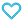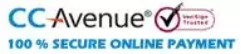# NCERT Text Book+Exemplar Problems Solutions Mathematics Class 11

250.00

Discount offer on this book in a bundle, click to view

MTG’s NCERT Textbook + Exemplar Problem solutions- Mathematics comprises of detailed and error free solutions of all the questions of NCERT Textbook and NCERT Exemplar Book. By practicing these questions students will develop analytical approach to solve problems.

862 in stock

Qty:Add to WishlistMTG’s NCERT Textbook + Exemplar Problem solutions- Mathematics is an excellent book designed to provide a comprehensive guide to NCERT Textbook and NCERT Exemplar Problems of Mathematics for Class XI students. This book provides a detailed explanation to all subjective and objective type questions given in both NCERT Textbook and NCERT Exemplar Problems book. This is an error free and ‘Self-study’ book to master the concepts in Mathematics.

This book aims to give the best solutions and help the students to score high in school examinations as well as to build a strong foundation for Olympiads and competitive exams like PETs & PMTs.

Table of Content:
• 1. Sets
• 2. Relations and Functions
• 3. Trigonometric Functions
• 4. Principle of Mathematical Induction
• 5. Complex Numbers and Quadratic Equations
• 6. Linear Inequalities
• 7. Permutations and Combinations
• 8. Binomial Theorem
• 9. Sequences and Series
• 10. Straight Lines
• 11. Conic Sections
• 12. Introduction to Three Dimensional Geometry
• 13. Limits and Derivatives
• 14. Mathematical Reasoning
• 15. Statistics
• 16. Probability
ISBN11 9789389167443 MTG Editorial Board 2019-20 356 Class 11 School Books Mathematics 452gm

## Reviews

There are no reviews yet.